Select Page

# Relations and Functions MCQ CBSE Maths 12 Science Answers in English

Relations and Functions MCQ CBSE Maths 12 Science Answers in English to enable students to get Answers in a narrative video format for the specific question.

Expert Teacher provides Relations and Functions MCQ CBSE Maths 12 Science Answers through Video Answers in English language. This video solution will be useful for students to understand how to write an answer in exam in order to score more marks. This teacher uses a narrative style for a question from Relations and Functions not only to explain the proper method of answering question, but deriving right answer too.

Please find the question below and view the Answer in a narrative video format.

Question:

## Similar Questions from CBSE, 12th Science, Maths, Relations and Functions

Question 1 : Number of binary sets on the set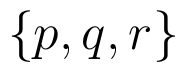is, (View Answer Video)

Question 2 :  Let * be any binary operation on the set R defined by a * b = a + b – ab, then the binary operation * is, (View Answer Video)

Question 3 : A functiondefined as f(x) = 5x is, (View Answer Video)

Question 4 : Letdefined as f(x) = x be an identity function. Then, (View Answer Video)

Question 5 : If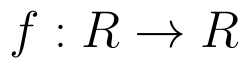is defined by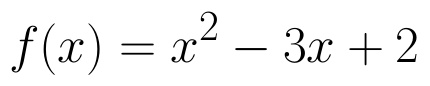, write f(f(x)). (View Answer Video)

### Application of Derivatives

Question 1 : The rate of change of the area of a circle with respect to its radius r at r=6cm is: (View Answer Video)

Question 2 : A cylindrical tank of radius 10 m is being filled with wheat at the rate of 314 cubic meters per hour. Then the depth of the wheat is increasing at the rate of: (View Answer Video)

Question 3 : Find the approximate change in the surface area of a cube of side x meters caused by decreasing the side by 1%. (View Answer Video)

Question 4 : The line y=mx+1 is a tangent to the curve if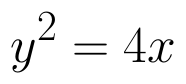the value of m is ________. (View Answer Video)

Question 5 : The slope of the tangent to the curve: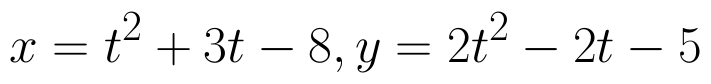### Application of Integrals

Question 1 : Using integration, find the area bounded by the tangent to the curve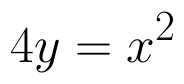at the point (2, 1) and the lines whose equations are x = 2y and x = 3y - 3. (View Answer Video)

Question 2 : Find the area of the region enclosed by the parabola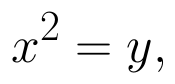the line y = x + 2. (View Answer Video)

Question 3 : Find the area of the region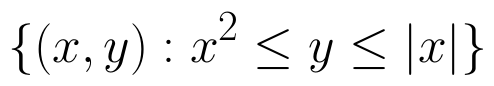. (View Answer Video)

Question 4 : Using integration, find the area of the region bounded by the triangle whose vertices are (-1, 2), (1, 5) and (3, 4). (View Answer Video)

Question 5 : Using integration, find the area of the region bounded by the line 2x + y = 4, 3x - 2y = 6 and x - 3y + 5 = 0. (View Answer Video)

### Inverse Trigonometric Functions

Question 1 :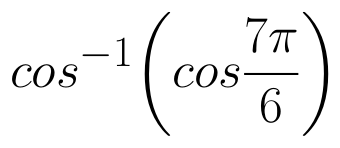is equal to :
Question 2 : If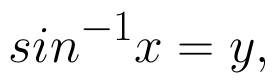then value of y lies between.....? (View Answer Video)
Question 3 :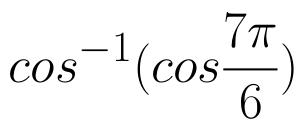is equal to : (View Answer Video)
Question 4 : If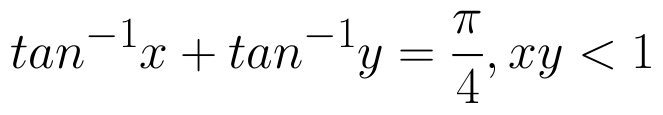, then write the value of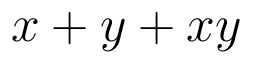. (View Answer Video)
Question 5 : Write the principal value of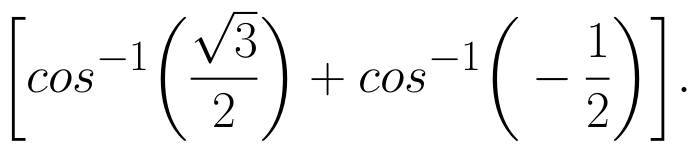(View Answer Video)